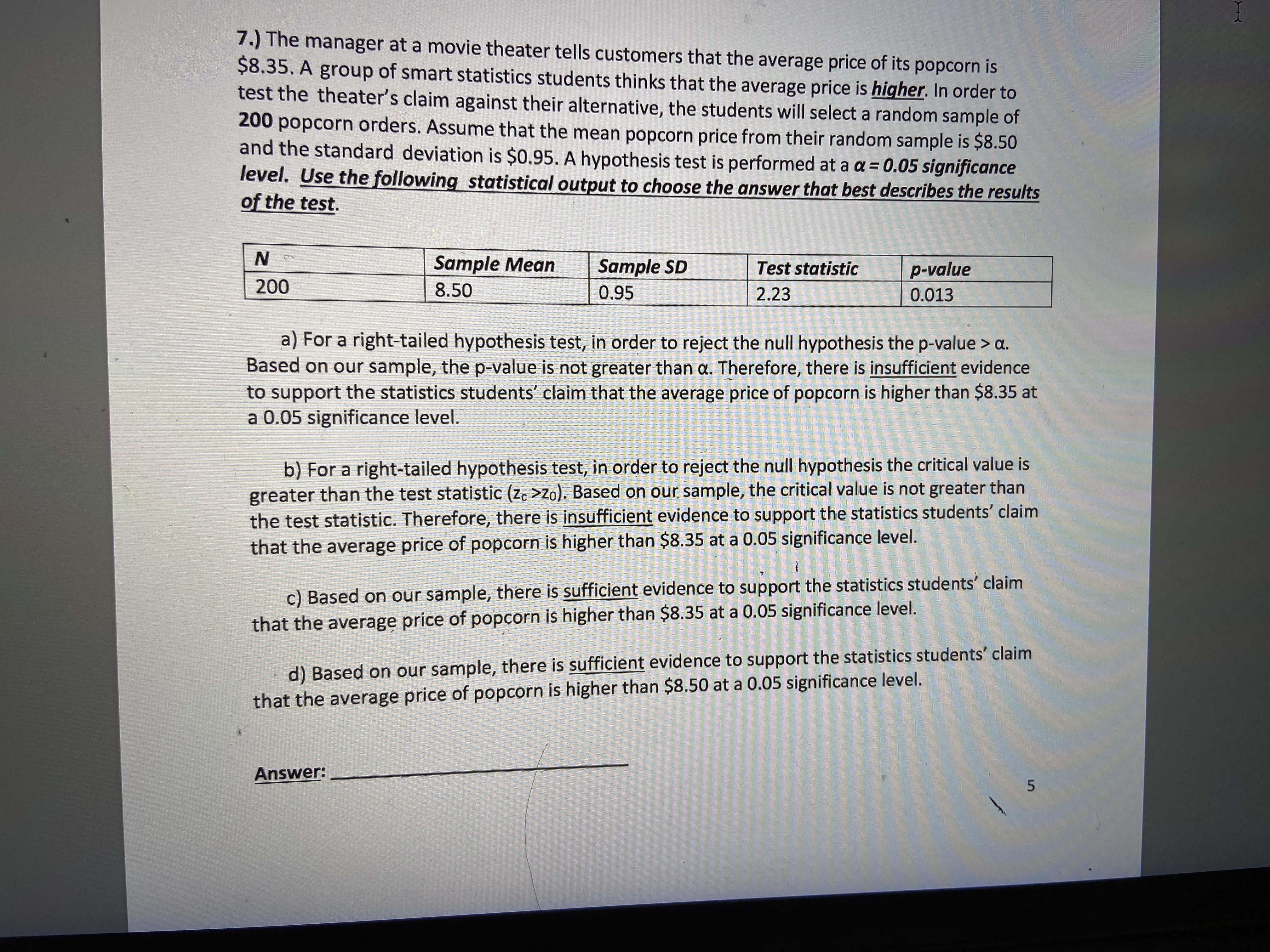# 7.) The manager at a movie theater tells customers that the average price of its popcorn is $8.35. A group of smart statistics students thinks that the average price is higher. In order to test the theater's claim against their alternative, the students will select a random sample of 200 popcorn orders. Assume that the mean popcorn price from their random sample is$8.50 and the standard deviation is $0.95. A hypothesis test is performed at a a = 0.05 significance level. Use the following statistical output to choose the answer that best describes the results of the test. Question using the following statistical output what wouod be the best answer that describes results on test?help_outlineImage Transcriptionclose7.) The manager at a movie theater tells customers that the average price of its popcorn is$8.35. A group of smart statistics students thinks that the average price is higher. In order to test the theater's claim against their alternative, the students will select a random sample of 200 popcorn orders. Assume that the mean popcorn price from their random sample is $8.50 and the standard deviation is$0.95. A hypothesis test is performed at a a = 0.05 significance level. Use the following statistical output to choose the answer that best describes the results of the test. fullscreen

### Want to see this answer and more?

Experts are waiting 24/7 to provide step-by-step solutions in as fast as 30 minutes!*

*Response times may vary by subject and question complexity. Median response time is 34 minutes for paid subscribers and may be longer for promotional offers.
Tagged in
Math
Statistics

### Hypothesis Testing